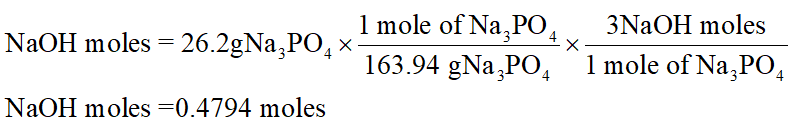# Determine the volume of NaOH solution needed to prepare 26.2 g of Na3PO4 by the reaction 3 NaOH + H3PO4 → Na3PO4 + 3 H2O The NaOH solution is 12% NaOH by mass and has a density of 1.133 g/mL. Show all steps clearly. Report the answer to the correct number of significant figures and with the appropriate units.

Question
72 views

Determine the volume of NaOH solution needed to prepare 26.2 g of Na3PO4 by the reaction

3 NaOH + H3PO4 → Na3PO4 + 3 H2O

The NaOH solution is 12% NaOH by mass and has a density of 1.133 g/mL. Show all steps clearly. Report the answer to the correct number of significant figures and with the appropriate units.

check_circle

Step 1

Observing the given balanced equation clearly shows that 1 mole of Na3PO4 is obtained from 3 moles of NaOH.

The moles of NaOH required is determined as shown below,Next molarity of NaOH is calculated.

...

### Want to see the full answer?

See Solution

#### Want to see this answer and more?

Solutions are written by subject experts who are available 24/7. Questions are typically answered within 1 hour.*

See Solution
*Response times may vary by subject and question.
Tagged in